# Set-20 Alphanumeric Series For SBI PO and SBI Clerk 2019 | Must Go Through These QuestionsDear Aspirants,
We are providing the most important  Alphanumeric Series Questions for SBI PO 2019, SBI Clerk 2019 and all other competitive bank and insurance exams. These questions have very high chances to be asked in SBI PO 2019, SBI Clerk 2019.
Get the Best Test Series for SBI PO 2019 at the most affordable price (Based on Real Exam Pattern) – Click Here
Download the Best GK Gaming App for Current Affairs and GK (Bank+SSC)– Click here (App No 1)       (App No 2)

Directions (1-5): Answer the following questions referring to the symbol-letter-number sequence given below: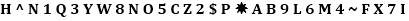1. How many symbols are there in the above sequence which are immediately preceded by a number and immediately followed by a consonant?

2. What should come in place of the question mark (?) in the following sequence?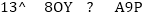3. Which of the following is exactly middle between the seventh element from the right end and sixth element from the left end?

4. Which of the following is the fifth element to the left of the ninth element from the right end in the above sequence?

5. If first and second halves of the above sequence are written in reverse order, then which will be the sixth element to the right of the fourteenth element from the right end?

Directions (6-10): Study the following arrangement carefully and answer the questions given below.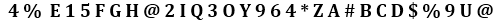6. How many vowels are immidiately preceeded by a number but not followed by a consonant?

7. How many consonents are preceeded by vowel &followed by a number?

8. Which element is 4th to the right of 12th element from the right end?

9. If Four of the following five are alike in a certain way and thus form a group. Which is the one that does not belong to that group?

10. If all the numbers are removed from the above series,then which element is 14th from the left end?

Check your Answers below:

Directions (1-5): Answer the following questions referring to the symbol-letter-number sequence given below:##### 1. Question

How many symbols are there in the above sequence which are immediately preceded by a number and immediately followed by a consonant?

Ans: 2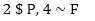##### 2. Question

What should come in place of the question mark (?) in the following sequence?Ans: 4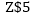##### 3. Question

Which of the following is exactly middle between the seventh element from the right end and sixth element from the left end?

Ans: 2
‘2’

##### 4. Question

Which of the following is the fifth element to the left of the ninth element from the right end in the above sequence?

Ans: 2
‘P’

##### 5. Question

If first and second halves of the above sequence are written in reverse order, then which will be the sixth element to the right of the fourteenth element from the right end?

Ans: 3
‘6’

##### 6. Question

Directions (6-10): Study the following arrangement carefully and answer the questions given below.How many vowels are immidiately preceeded by a number but not followed by a consonant?

Ans: 3
9U@

##### 7. Question

How many consonents are preceeded by vowel &followed by a number?

Ans: 1
IQ3 ,OY9

##### 8. Question

Which element is 4th to the right of 12th element from the right end?

Ans: 1
B

##### 9. Question

If Four of the following five are alike in a certain way and thus form a group. Which is the one that does not belong to that group?

Ans: 3
\$UD

##### 10. Question

If all the numbers are removed from the above series,then which element is 14th from the left end?

Ans: 4
##### About Study Virus

View all posts by Study Virus →

This site uses Akismet to reduce spam. Learn how your comment data is processed.